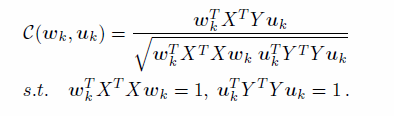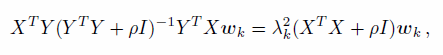## Iterative Quantization

IterativeQuantization: A Procrustean Approach to Learning Binary Codes 论文理解及代码讲解

（1）     用短的二值码来代图像，可以在内存中存储大量的图像。

（2）     比较两幅图像相似性，用二值码来计算hamming距离，非常快速，高校。

（3）     用这个方法可以代替大规模图像索引方法。

（1）     编码要短。（16G的内存，存储250million图像在内存里的话，对于每图像只能使用64bits

（2）     图像映射到二值码后，两个编码要尽可能的相似，也就是原来两幅图像距离大，映射到二值码后，hamming距离也很大。

（3）     用来学习二值码的算法要足够的高效，并且对新的图像编码成二值码要efficient

# ITQ的思想：

（1）     PCA对原始数据进行降维（如果有监督则用CCA来降维）

（2）     然后把把原始数据分别映射到超立方体的顶点，因为超立方体的顶点是二值码，求解量化误差最小的映射方式。量化二值码的方法是：hk(x) = sgn(xwk)，用sgn函数来量化。

（3）     本文的创新点是对这个超立方体在空间中旋转，然后求解初旋转矩阵就可以得到最好的映射方式。

（注意：文章不是直接对超立方体进行旋转，而是对数据进行旋转后，再映射到立方体上，相当于物理上运动的相对性，数据旋转，立方体不动，可以看成数据不动，立方体在旋转，一样的道理，文章为了方便求解旋转矩阵，对数据进行旋转。）

# 对于unsupervised code learning

ITQ 步骤可以简答的概括为（将原始d维向量映射到c维的二值码）：

1）假设数据是centralized，如果不是，用程序进行centralizedX是原始数据（n*d维矩阵），中心化的matlab代码：

sample_mean = mean(X, 1);

X = bsxfun(@minus, X,sample_mean);

2）将原始数据做一个PCA投影，这一步主要是为了降维。具体PCA降维过程不做过多赘述。

covX = X' * X / size(X,1);

OPTS.disp = 0;

[eigVec, eigVal] =eigs(double(covX), num_bits, 'LM', OPTS);

X = X * eigVec;

（求解离散度矩阵的特征值，选出前C个最大特征值对应的特征向量做为投影矩阵，这样就把原始数据降维到c维）

（3）二值量化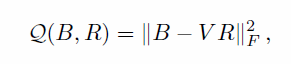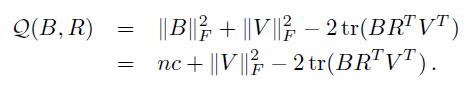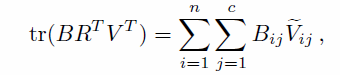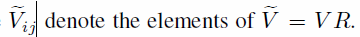（1）    固定R,求解B

R = randn(bit,bit);  // 随机生成一个矩阵

[U11 S2 V2] = svd(R); //对矩阵做SVD分解，U和V都是正交矩阵

R = U11(:,1:bit);   //选取U作为随机初始化的正交矩阵R

R固定了，求解B相当简单。最大化（2）      固定B,求解R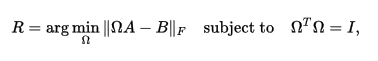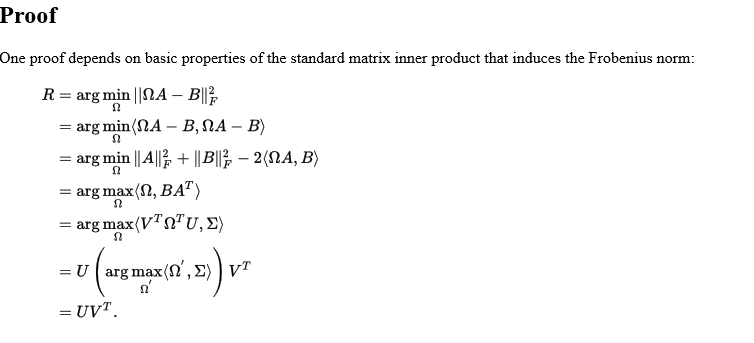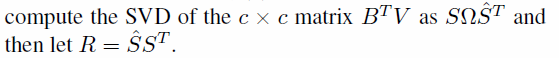% ITQ to find optimal rotation

for iter=0:n_iter

if mod(iter,10)==0

fprintf('ITQ: iter: %d \n', iter);

end

Z = V * R;

UX = ones(size(Z,1),size(Z,2)).*-1;

UX(Z>=0) = 1;

C = UX' * V;

[UB,sigma,UA] = svd(C);

R = UA * UB';

end

% make B binary

B = UX;

B(B<0) = 0;

BincodeXtrainingITQ =compactbit(bsxfun(@minus, Xtraining, sample_mean) * eigVec * R > 0);

# 利用label信息的ITQ二值码的映射：

CCA 的英文全称是Canonical Correlation Analysis，目标是找到特征的投影W矩阵和标签的投影矩阵U，是的投影后的XW和YU的相关性最大。